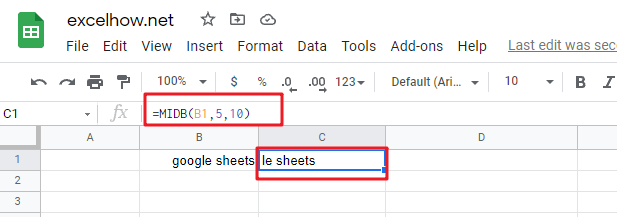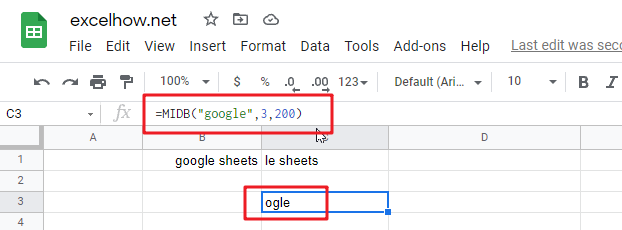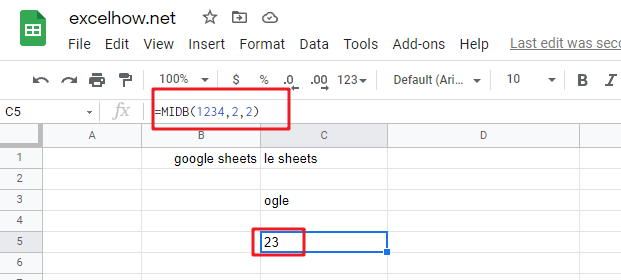# Google Sheets MIDB Function

This post will guide you how to use Google Sheets MIDB function with syntax and examples.

## Description

The Google Sheets MIDB function returns a substring (a specified number of bytes) starting from the middle of a text string and up to a specified number of bytes.

The MIDB function can be used to extract a given bytes of characters from the middle of a text string in google sheets. It returns a substring from a text string at the position that you specify.

The MIDB function is a build-in function in Google Sheets and it is categorized as a Text Function.

## Syntax

The syntax of the MIDB function is as below:

= MIDB (text, start_num, length_bytes)

Where the MIDB function arguments are:

• text -This is a required argument. The text string that you want to extract substring from.
• start_num-This is a required argument.  The position of the first character that you want to extract in text string. The index from the left of text string from which to begin extracting. And the first character in text string is the index 1.
• length_bytes-This is a required argument.  The number of the bytes that you want to extract from a text string.

Note:

• If `start_num`` `is greater than the length of text, the MIDB function will return empty text.
• If `start_num` is less than 1, the MIDB function will return the #VALUE! Error value.
• If `Length_bytesis` negative, MIDB will return the #VALUE!  Error value.
• If you want to extract a substring from start_num to the end of text string, and you can use the LEN function to calculate the length of the text string, you should not specify a large number to `length_bytes` argument for this case.
• If you want to extract a substring beginning with a particular character, and you should use the SEARCH function to get the index of  that character in text string.
• `Length_bytes` must be greater than or equal to 0.
• `Start_num` must be greater than or equal to 1.
• If text string has only single bytes characters and the MIDB function returns the same value as MID function.

## Google Sheets MIDB Function Examples

The below examples will show you how to use google sheets MIDB Text function to extract a substring from a text string.

#1 To extract 10 bytes of substring  from the text string in B1, starting at the 5th character, just using the following formula:

`=MIDB(B1,5,10)  //it returns “le sheets”`#2 If num_chars value is greater than the length of remaining bytes, and the MID function will return all remining characters:

`=MIDB("google",3,200) //it returns “ogle”`#3 if text value is a number, and the returned value is also a text by MID function:

`=MIDB(1234,2,2)   //it returns “23”`Note:

You can use the LEFTB function to extract substring from the left side of a text string in Google Sheets. And you can also use another function called RIGHTB function to extract a substring starting from the right side of the text string. And if you wish to extract a substring starting from the middle position of text string, you can use the MIDB function in google sheets.

## Related Functions

• Google Sheets LEFT Function
The Google Sheets LEFT function returns a substring (a specified number of the characters) from a text string, starting from the leftmost character.The syntax of the LEFT function is as below:= LEFT(text,[num_chars])…
• Google Sheets LEN Function
The Google Sheets LEN function returns the length of a text string (the number of characters in a text string).The syntax of the LEN function is as below:= LEN(text)…

Related Posts

Google Sheets DEGREES Function

This post will guide you how to use Google Sheets DEGREES function with syntax and examples. Description The Google Sheets DEGREES function converts radians into degrees. And it will return a numeric value. For examples, if you pass the PI() function into ...

Google Sheets COSH Function

This post will guide you how to use Google Sheets COSH function with syntax and examples. Description The Google Sheets COSH function returns the hyperbolic cosine of a given angle. The purpose of this function is used to get hyperbolic ...

Google Sheets COS Function

This post will guide you how to use Google Sheets COS function with syntax and examples. Description The Google Sheets COS function returns the cosine of a given angle. If you want to supply an angle to COS function in ...

Google Sheets COMBINA Function

This post will guide you how to use Google Sheets COMBINA function with syntax and examples. Description The Google Sheets COMBINA function returns the number of combinations for a given number of items and it includes repetitions. You can use ...

Google Sheets COMBIN Function

This post will guide you how to use Google Sheets COMBIN function with syntax and examples. Description The Google Sheets COMBIN function returns the number of combinations for a given number of items. And you can use the COMBIN function ...

Sidebar